# Shading Ming Ouhyoung Professor Dept of CSIE and

• Slides: 86
Download presentation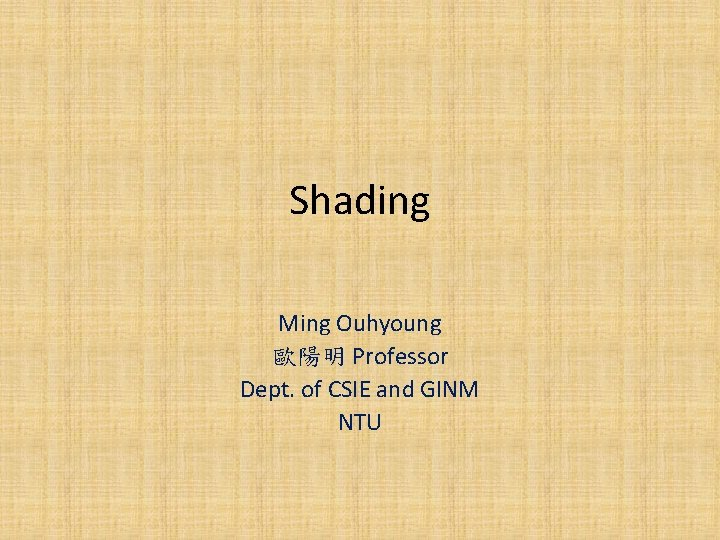Shading Ming Ouhyoung 歐陽明 Professor Dept. of CSIE and GINM NTUIllumination model 1) Ambient light (漫射) I = la * ka * Obj(r, g, b) la : intensity of ambient light ka : 0. 0 ~ 1. 0, Obj(r, g, b): object color 2) Diffuse reflection (散射) I = Ip (r, g, b) * Kd * Obj(r, g, b) * COS(theta-angle) Ip (r, g, b): light color 3) Light source attenuationSpecular reflection (似鏡面反射) • I = Ks * Ip(r, g, b) * COSn(alpha-angle), Ks = specular-reflection coef. • Phong illumination model (alpha=>反射光跟視角夾角) color of object = Obj (R, G, B) = (Or, Og, Ob) or (light frequency) where 0. 0 =< Od =< 1. 0 ( half way vector (近似, 快)=>(V+L)/2當做R )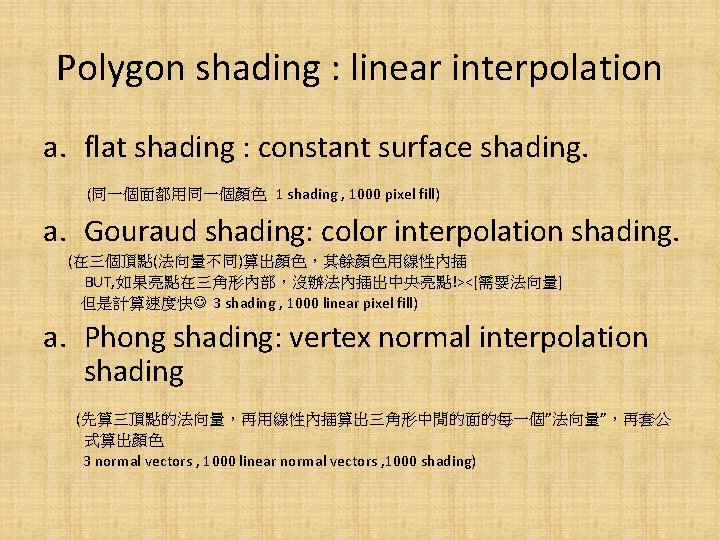Polygon shading : linear interpolation a. flat shading : constant surface shading. (同一個面都用同一個顏色 1 shading , 1000 pixel fill) a. Gouraud shading: color interpolation shading. (在三個頂點(法向量不同)算出顏色，其餘顏色用線性內插 BUT, 如果亮點在三角形內部，沒辦法內插出中央亮點!><[需要法向量] 但是計算速度快 3 shading , 1000 linear pixel fill) a. Phong shading: vertex normal interpolation shading (先算三頂點的法向量，再用線性內插算出三角形中間的面的每一個”法向量”，再套公 式算出顏色 3 normal vectors , 1000 linear normal vectors , 1000 shading)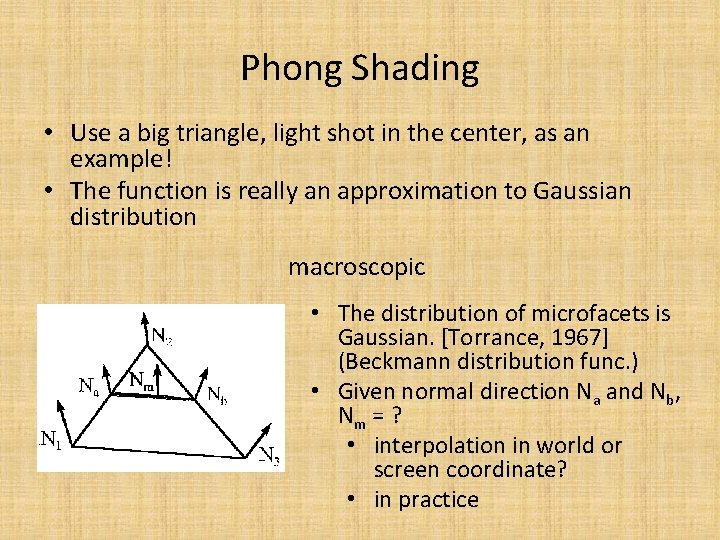Phong Shading • Use a big triangle, light shot in the center, as an example! • The function is really an approximation to Gaussian distribution macroscopic • The distribution of microfacets is Gaussian. [Torrance, 1967] (Beckmann distribution func. ) • Given normal direction Na and Nb, Nm = ? • interpolation in world or screen coordinate? • in practice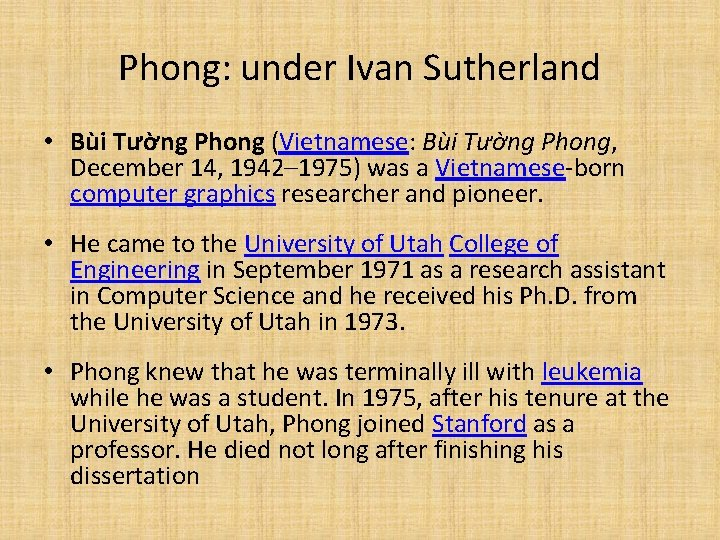Phong: under Ivan Sutherland • Bùi Tường Phong (Vietnamese: Bùi Tường Phong, December 14, 1942– 1975) was a Vietnamese-born computer graphics researcher and pioneer. • He came to the University of Utah College of Engineering in September 1971 as a research assistant in Computer Science and he received his Ph. D. from the University of Utah in 1973. • Phong knew that he was terminally ill with leukemia while he was a student. In 1975, after his tenure at the University of Utah, Phong joined Stanford as a professor. He died not long after finishing his dissertation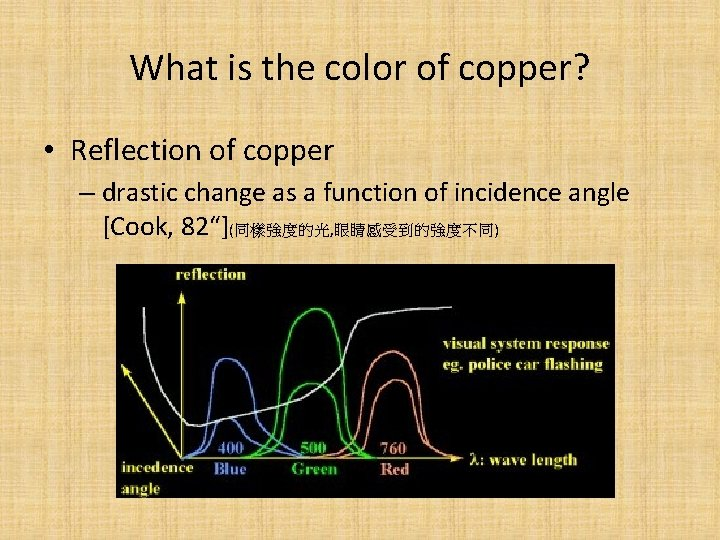What is the color of copper? • Reflection of copper – drastic change as a function of incidence angle [Cook, 82“](同樣強度的光, 眼睛感受到的強度不同)New method: BRDF: Bi-directional Reflectance Density Function • Use a camera to get the reflection of materials from many angles • Light is also from many anglesHomework #1 • Input : a file of polygons (triangles) • test image : a teapot, a tube • input format : Triangle fr, fg, fb, br, bg, bb x y z nx ny nz x 1, y 1, z 1, …. , , X 2, y 2, z 2 ……. , /* where (fr, fg, fb) contains front face colors, (br, bg, bb) are background colors (x, y, z): 3 D vertex position (nx, ny, nz) : vertex normal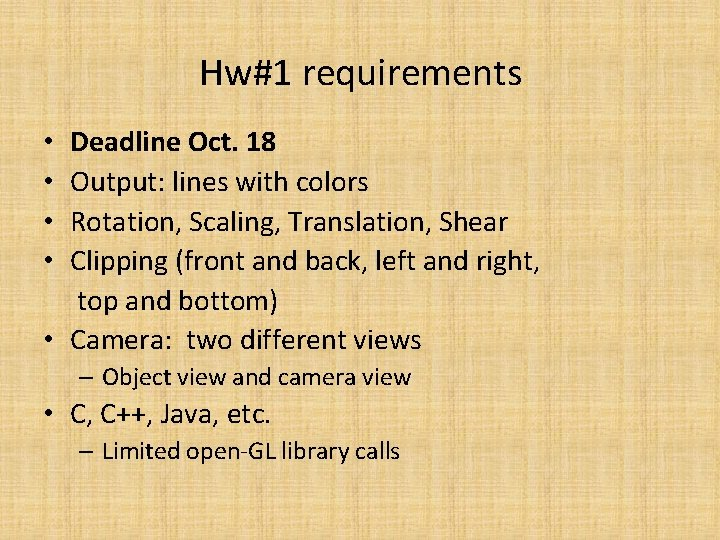Hw#1 requirements • Deadline Oct. 18 • Output: lines with colors • Rotation, Scaling, Translation, Shear • Clipping (front and back, left and right, top and bottom) • Camera: two different views – Object view and camera view • C, C++, Java, etc. – Limited open-GL library calls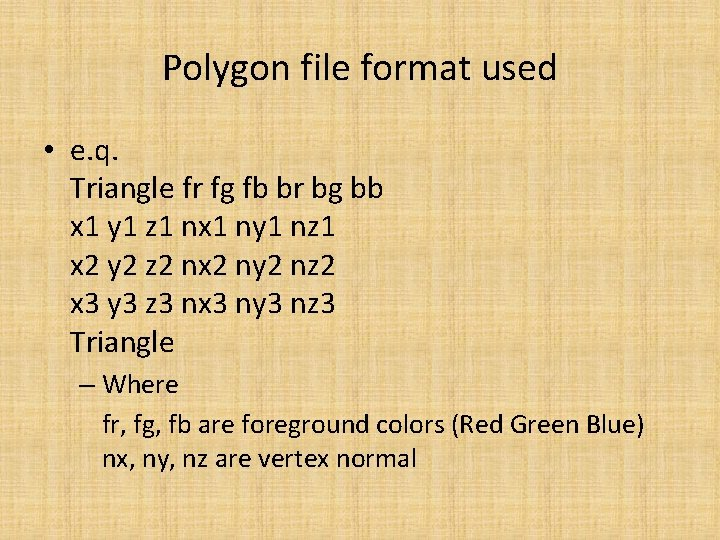Polygon file format used • e. q. Triangle fr fg fb br bg bb x 1 y 1 z 1 nx 1 ny 1 nz 1 x 2 y 2 z 2 nx 2 ny 2 nz 2 x 3 y 3 z 3 nx 3 ny 3 nz 3 Triangle – Where fr, fg, fb are foreground colors (Red Green Blue) nx, ny, nz are vertex normal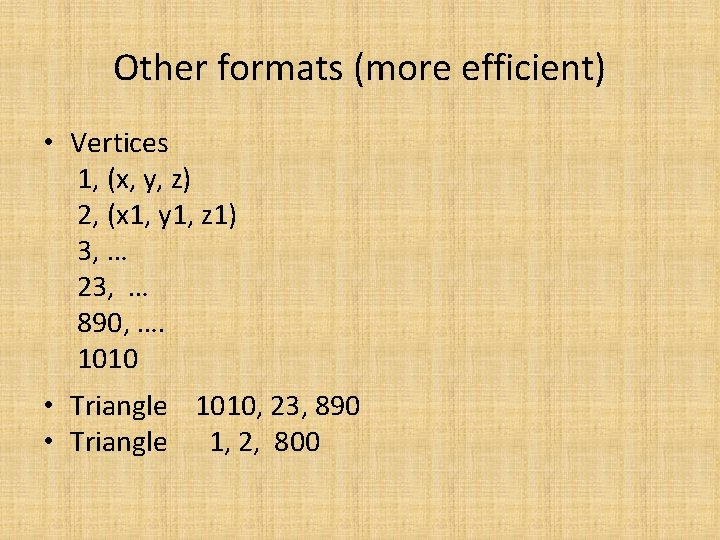Other formats (more efficient) • Vertices 1, (x, y, z) 2, (x 1, y 1, z 1) 3, … 23, … 890, …. 1010 • Triangle 1010, 23, 890 • Triangle 1, 2, 800Visible-Surface Determination • The painter‘s algorithm (由遠畫到近，蓋掉遠的[交叉圖形會出包]) • The Z-buffer algorithm (較晚出現 , use hardware to implement) – The point nearest to the eye is visible, . . . – Very easy both for software and hardware. – Hardware Implementation: Parallel ---> fast display • Scan-line algorithms – One scan line at a time • Area-subdivision algorithm – Divide and conquer strategy • Visible-surface ray tracing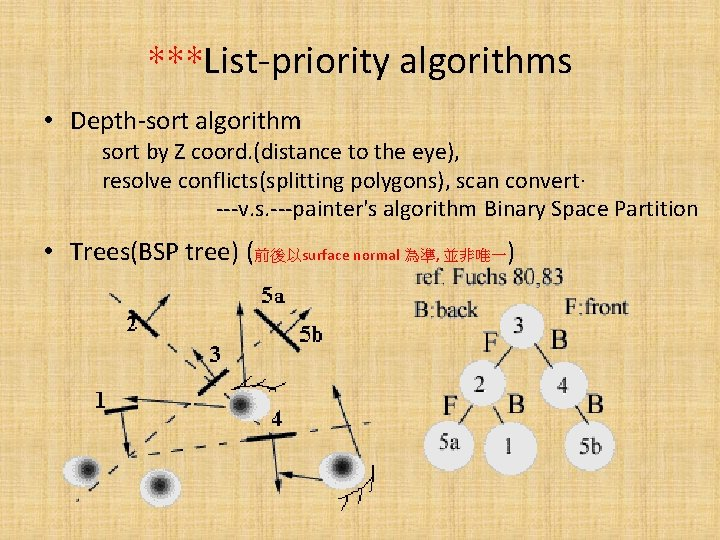***List-priority algorithms • Depth-sort algorithm sort by Z coord. (distance to the eye), resolve conflicts(splitting polygons), scan convert· ---v. s. ---painter's algorithm Binary Space Partition • Trees(BSP tree) (前後以surface normal 為準, 並非唯一)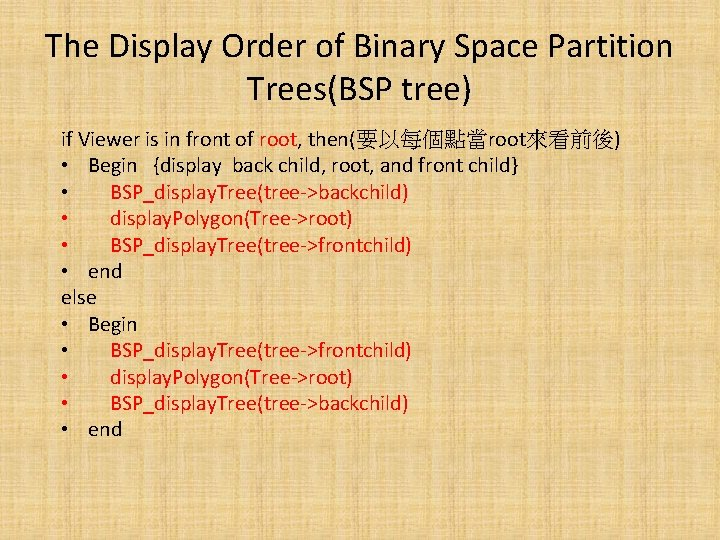The Display Order of Binary Space Partition Trees(BSP tree) if Viewer is in front of root, then(要以每個點當root來看前後) • Begin {display back child, root, and front child} • 　BSP_display. Tree(tree->backchild) • 　display. Polygon(Tree->root) • 　BSP_display. Tree(tree->frontchild) • end else • Begin • 　BSP_display. Tree(tree->frontchild) • 　display. Polygon(Tree->root) • 　BSP_display. Tree(tree->backchild) • end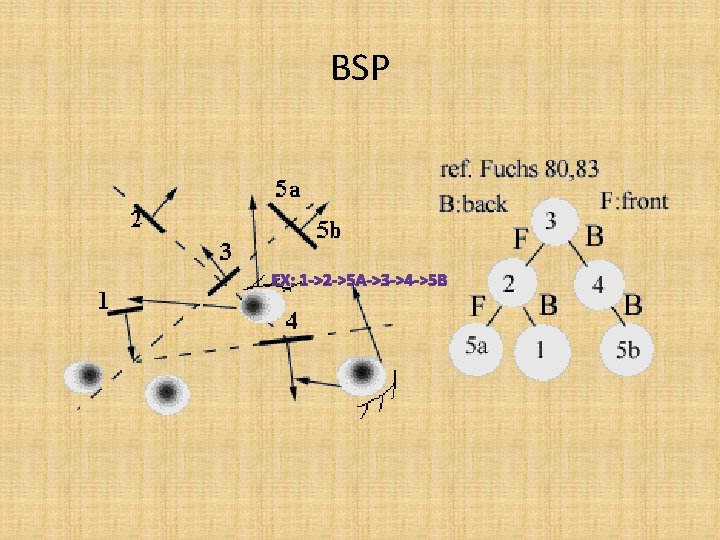BSP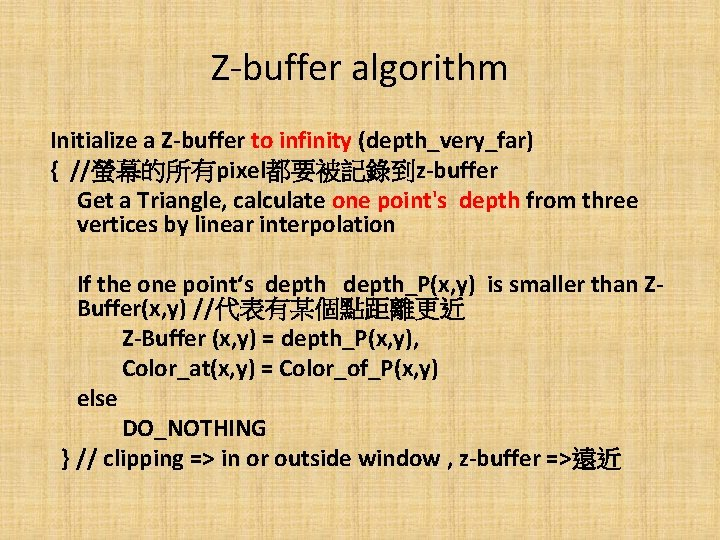Z-buffer algorithm Initialize a Z-buffer to infinity (depth_very_far) { //螢幕的所有pixel都要被記錄到z-buffer Get a Triangle, calculate one point's depth from three vertices by linear interpolation If the one point‘s depth_P(x, y) is smaller than ZBuffer(x, y) //代表有某個點距離更近 Z-Buffer (x, y) = depth_P(x, y), Color_at(x, y) = Color_of_P(x, y) else DO_NOTHING } // clipping => in or outside window , z-buffer =>遠近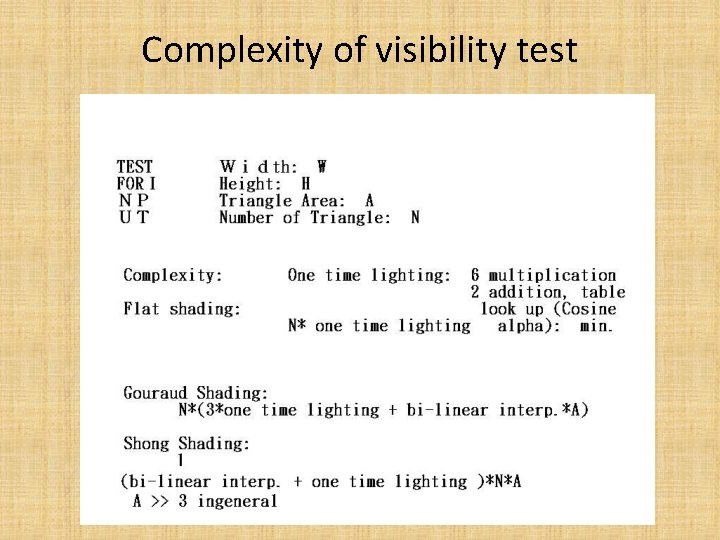Complexity of visibility test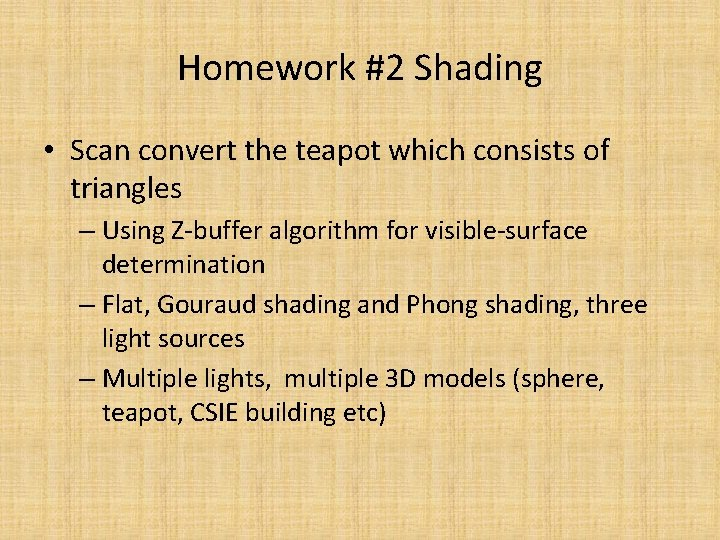Homework #2 Shading • Scan convert the teapot which consists of triangles – Using Z-buffer algorithm for visible-surface determination – Flat, Gouraud shading and Phong shading, three light sources – Multiple lights, multiple 3 D models (sphere, teapot, CSIE building etc)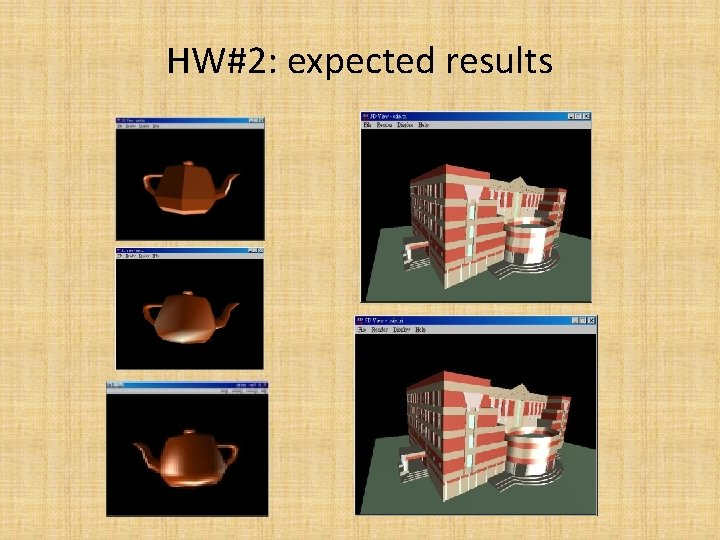HW#2: expected resultsHW#2: formula • – Ia is object color (ambient light 跟光源無關 不用一直算) – Ip is the color of light, and can have multiple lights • Note – color overflow problem (integer color up to 255) – MAX = max(R, G, B) = 255 etc. • Output format – RGBx. . 256*256 pixels – better results: 32<=R, G, B<=230, each 1 byte binary data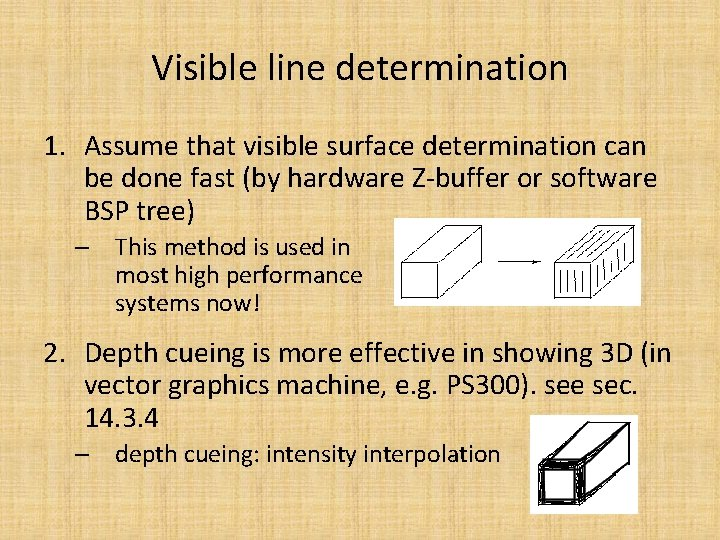Visible line determination 1. Assume that visible surface determination can be done fast (by hardware Z-buffer or software BSP tree) – This method is used in most high performance systems now! 2. Depth cueing is more effective in showing 3 D (in vector graphics machine, e. g. PS 300). see sec. 14. 3. 4 – depth cueing: intensity interpolation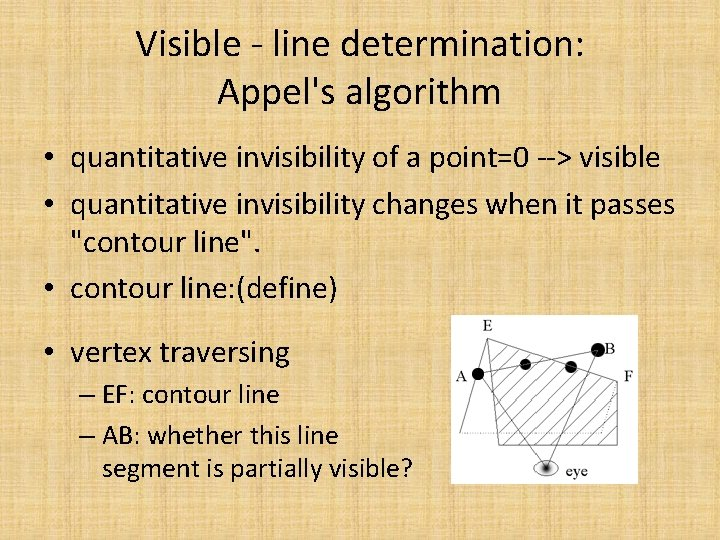Visible - line determination: Appel's algorithm • quantitative invisibility of a point=0 --> visible • quantitative invisibility changes when it passes "contour line". • contour line: (define) • vertex traversing – EF: contour line – AB: whether this line segment is partially visible?Standard Graphics Pipeline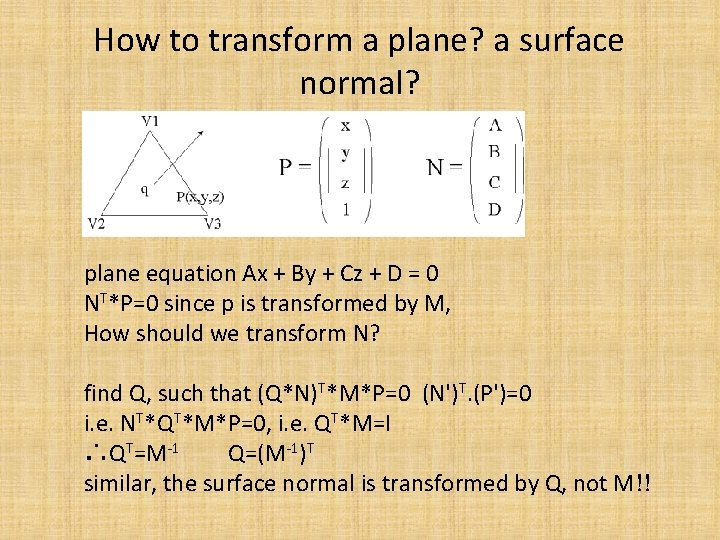How to transform a plane? a surface normal? plane equation Ax + By + Cz + D = 0 NT*P=0 since p is transformed by M, How should we transform N? find Q, such that (Q*N)T*M*P=0 (N')T. (P')=0 i. e. NT*QT*M*P=0, i. e. QT*M=I ∴QT=M-1 Q=(M-1)T similar, the surface normal is transformed by Q, not M!!Aliasing, anti-aliasingAnti-aliasing results: sharp lines and triangles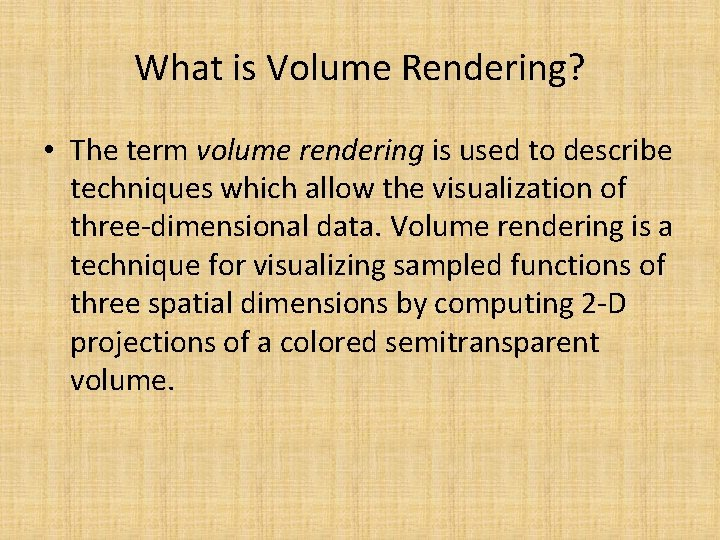What is Volume Rendering? • The term volume rendering is used to describe techniques which allow the visualization of three-dimensional data. Volume rendering is a technique for visualizing sampled functions of three spatial dimensions by computing 2 -D projections of a colored semitransparent volume.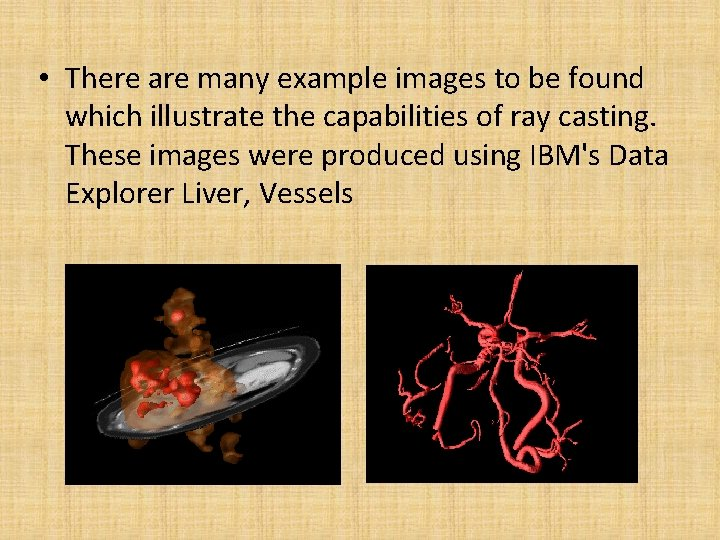• There are many example images to be found which illustrate the capabilities of ray casting. These images were produced using IBM's Data Explorer Liver, Vessels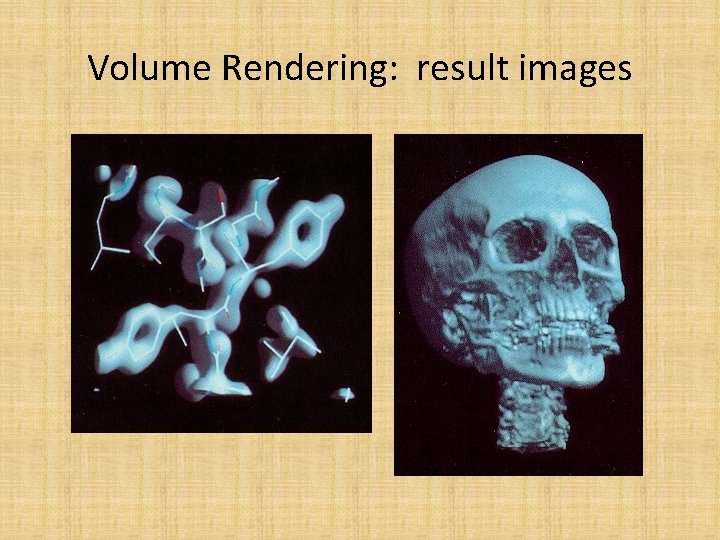Volume Rendering: result images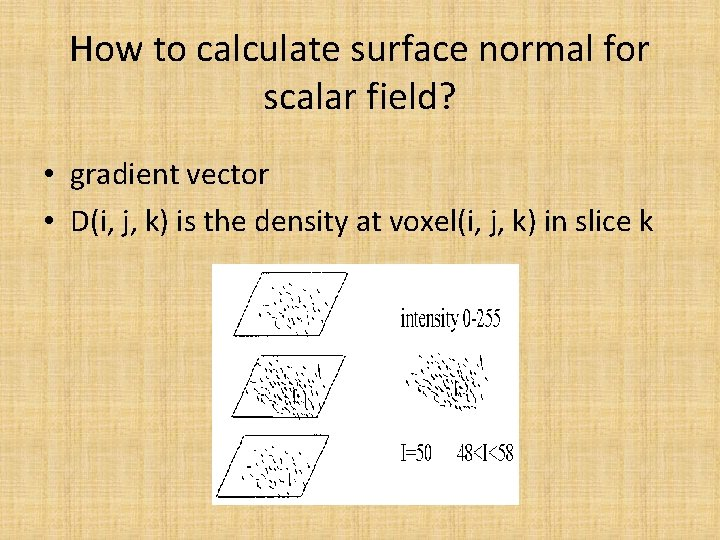How to calculate surface normal for scalar field? • gradient vector • D(i, j, k) is the density at voxel(i, j, k) in slice kMarching cubes (squares)Ray casting for volume rendering • Theory – Currently, most volume rendering that uses ray casting is based on the Blinn/Kajiya model. In this model we have a volume which has a density D(x, y, z), penetrated by a ray R.• Rays are cast from the eye to the voxel, and the values of C(X) and (X) are "combined" into single values to provide a final pixel intensity.Transparency formula • For a single voxel along a ray, the standard transparency formula is: where: – Cout is the outgoing intensity/color for voxel X along the ray – Cinis the incoming intensity for the voxel • Splatting for transparent objects: back to front rendering – Eye Destination Voxel Source Voxel – Cd’= (1 -αs) Cd +αs Cs αd’= (1 -αs) αd + αs Cs : Color of source (background object color) αs: Opaque index (opaque = 1. 0, transparency = 0. 0) when background αs = 1. 0, destination αd = 0. 0, Cd’ = Cs, αd’ =αs, similarly, when foreground (destination) is NOT transparent, αd = 1. 0, Cd’ = Cd (color of itself)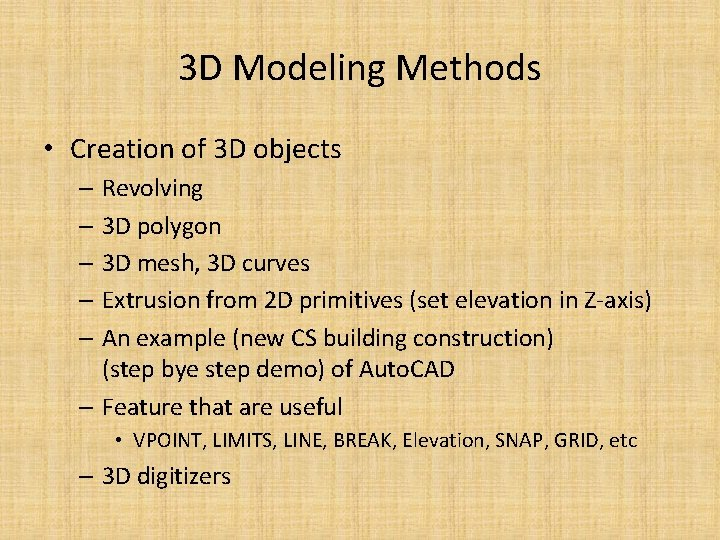3 D Modeling Methods • Creation of 3 D objects – Revolving – 3 D polygon – 3 D mesh, 3 D curves – Extrusion from 2 D primitives (set elevation in Z-axis) – An example (new CS building construction) (step bye step demo) of Auto. CAD – Feature that are useful • VPOINT, LIMITS, LINE, BREAK, Elevation, SNAP, GRID, etc – 3 D digitizersTexture mapping 1. What is texture? 2. How to map a texture to an object surface? <- : direction of mapping pixel value = sum of weighted texels within the four corners mapped from a pixel 3. See pictures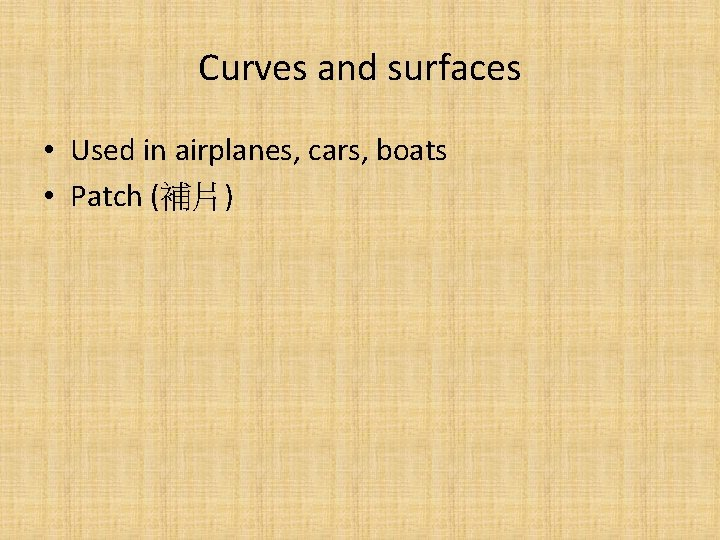Curves and surfaces • Used in airplanes, cars, boats • Patch (補片)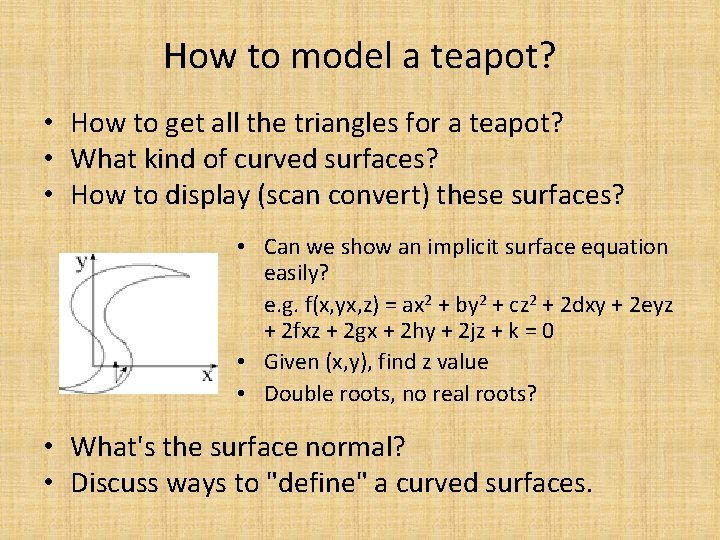How to model a teapot? • How to get all the triangles for a teapot? • What kind of curved surfaces? • How to display (scan convert) these surfaces? • Can we show an implicit surface equation easily? e. g. f(x, yx, z) = ax 2 + by 2 + cz 2 + 2 dxy + 2 eyz + 2 fxz + 2 gx + 2 hy + 2 jz + k = 0 • Given (x, y), find z value • Double roots, no real roots? • What's the surface normal? • Discuss ways to "define" a curved surfaces.Curves and Surfaces • Topics – – Polygon meshes Parametric cubic curves Parametric bicubic surfaces Quadric surfaces Parametric cubic curves x(t) = axt 3 + bxt 2 + cxt + dx y(t) = ayt 3 + byt 2 + cyt + dy z(t) = azt 3 + bzt 2 + czt + dz • Continuity conditions – Geometric continuity (G 0): join together – Parametric continuity (C 1) (see below) – Cncontinuity: dn / dtn[Q(t)]continuous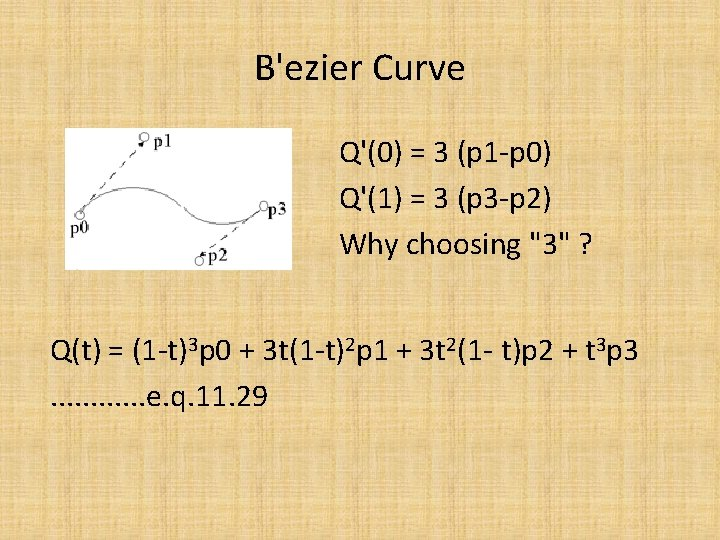B'ezier Curve Q'(0) = 3 (p 1 -p 0) Q'(1) = 3 (p 3 -p 2) Why choosing "3" ? Q(t) = (1 -t)3 p 0 + 3 t(1 -t)2 p 1 + 3 t 2(1 - t)p 2 + t 3 p 3. . . e. q. 11. 29Bezier curve(2) in matrix form T*MB*GB Note: Q'(0) = -3(1 -t)2 p 0 + 3(1 -t)2 p 1|t=0 =3(p 1 -p 0) if p 1 - p 4 is equally spaced, the curve Q(t) has constant velocity! (that's why to choose 3)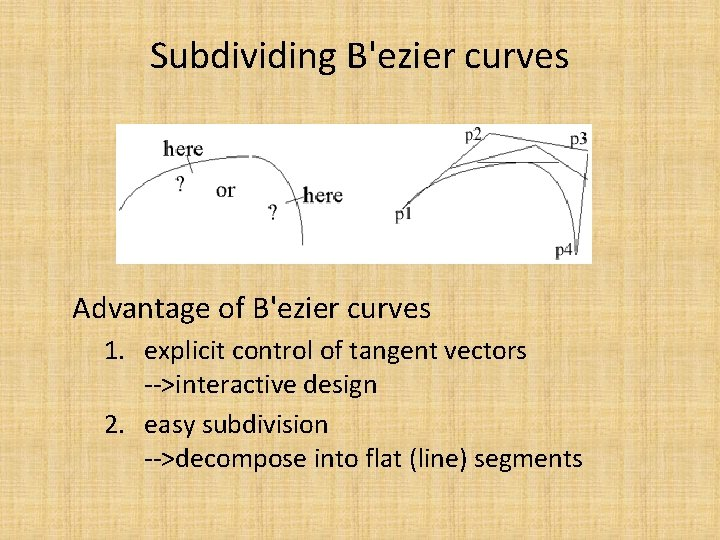Subdividing B'ezier curves Advantage of B'ezier curves 1. explicit control of tangent vectors -->interactive design 2. easy subdivision -->decompose into flat (line) segments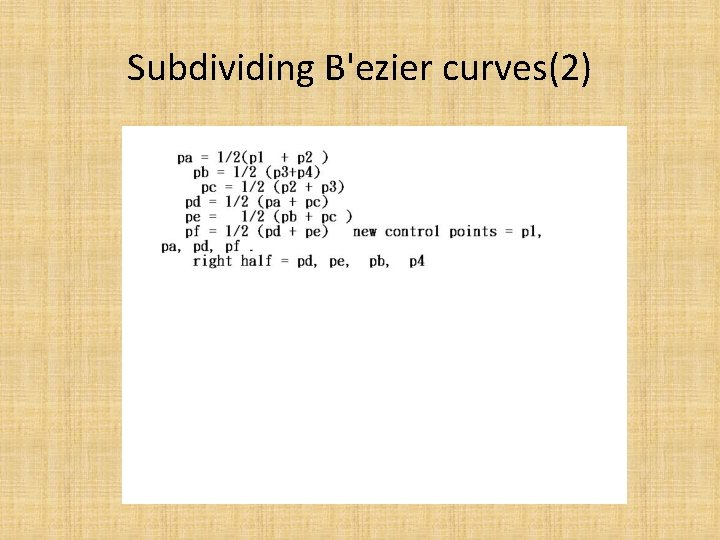Subdividing B'ezier curves(2)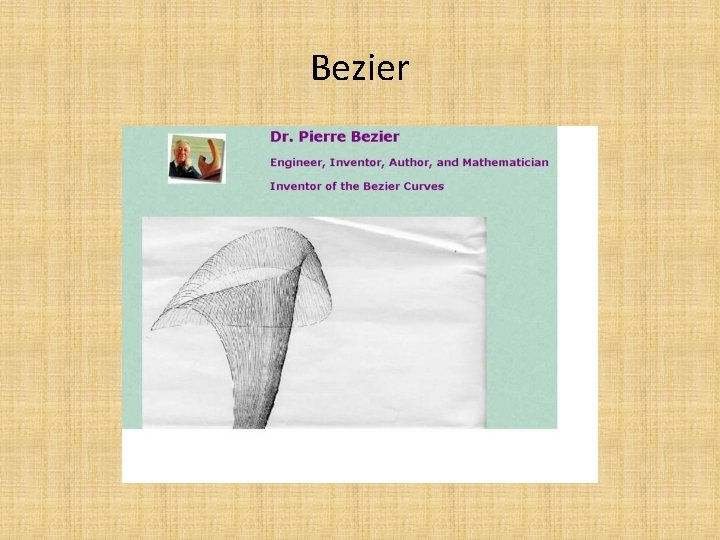BezierPierre Etienne B’ezier Introduction • Pierre Etienne Bezier was born on September 1, 1910 in Paris. Son and grandson of engineers, he chose this profession too and enrolled to study mechanical engineering at the Ecole des Arts et Metiers and received his degree in 1930. In the same year he entered the Ecole Superieure d'Electricite and earnt a second degree in electrical engineering in 1931. In 1977, 46 years later, he received his DSc degree in mathematics from the University of Paris.In 1933, aged 23, Bezier entered Renault and worked for this company for 42 years • Bezier's academic career began in 1968 when he became Professor of Production Engineering at the Conservatoire National des Arts et Metiers. He held this position until 1979. He wrote four books, numerous papers and received several distinctions including the "Steven Anson Coons" of the Association for Computing Machinery and the "Doctor Honoris Causa" of the Technical University Berlin. He is an honorary member of the American Society of Mechanical Engineers and of the Societe Belge des Mecaniciens, ex-president of the Societe des Ingenieurs et Scientifiques de France, Societe des Ingenieurs Arts et Metiers, and he was one of the first Advisory Editors of "Computer-Aided Design".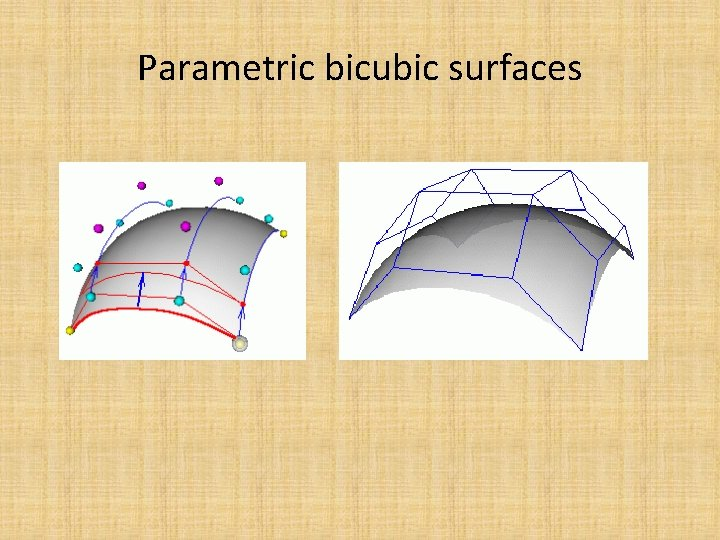Parametric bicubic surfaces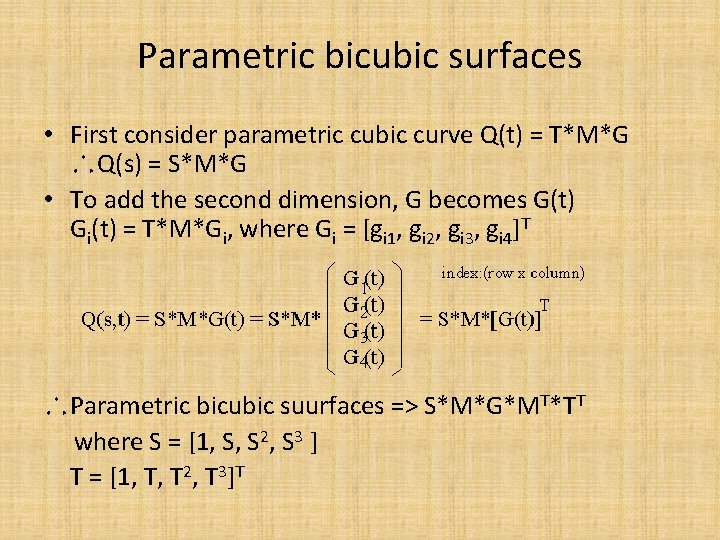Parametric bicubic surfaces • First consider parametric cubic curve Q(t) = T*M*G ∴Q(s) = S*M*G • To add the second dimension, G becomes G(t) Gi(t) = T*M*Gi, where Gi = [gi 1, gi 2, gi 3, gi 4]T ∴Parametric bicubic suurfaces => S*M*G*MT*TT where S = [1, S, S 2, S 3 ] T = [1, T, T 2, T 3]T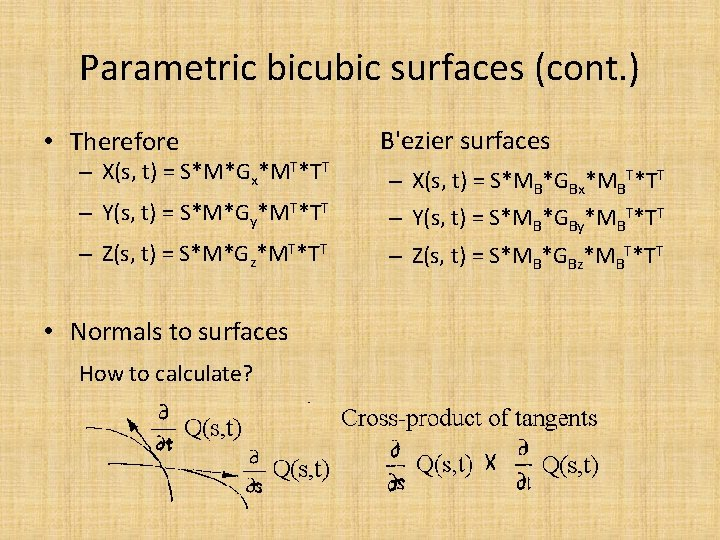Parametric bicubic surfaces (cont. ) • Therefore – X(s, t) = S*M*Gx*MT*TT – Y(s, t) = S*M*Gy*MT*TT – Z(s, t) = S*M*Gz*MT*TT • Normals to surfaces How to calculate? B'ezier surfaces – X(s, t) = S*MB*GBx*MBT*TT – Y(s, t) = S*MB*GBy*MBT*TT – Z(s, t) = S*MB*GBz*MBT*TT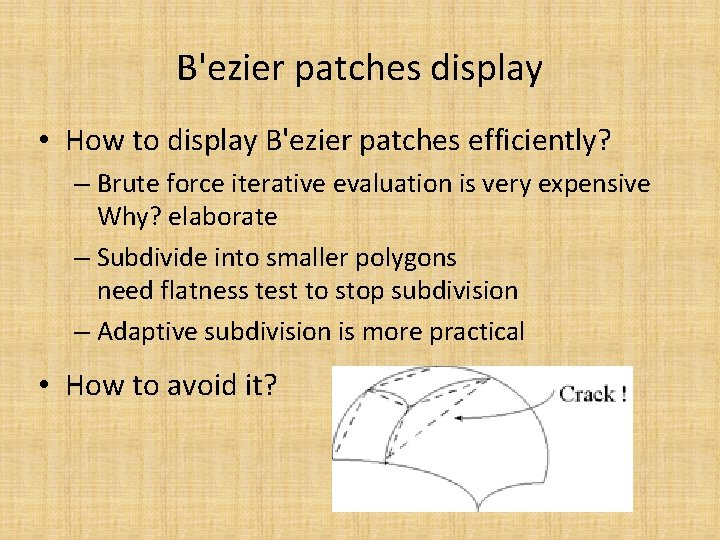B'ezier patches display • How to display B'ezier patches efficiently? – Brute force iterative evaluation is very expensive Why? elaborate – Subdivide into smaller polygons need flatness test to stop subdivision – Adaptive subdivision is more practical • How to avoid it?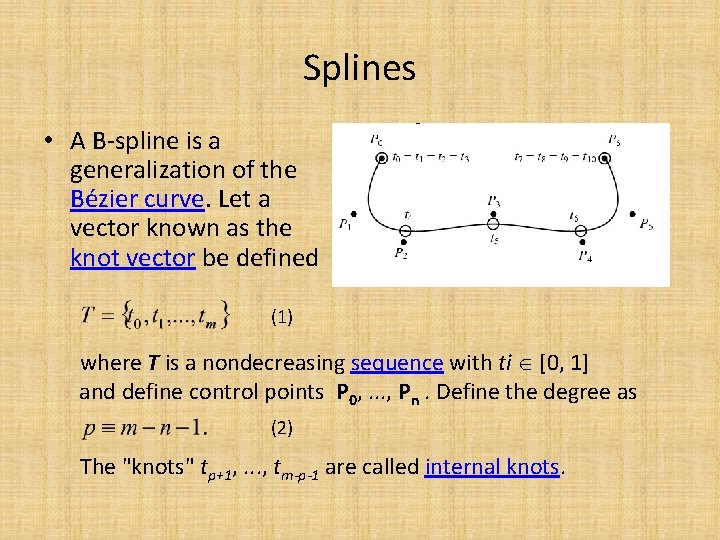Splines • A B-spline is a generalization of the Bézier curve. Let a vector known as the knot vector be defined (1) where T is a nondecreasing sequence with ti [0, 1] and define control points P 0, . . . , Pn. Define the degree as (2) The "knots" tp+1, . . . , tm-p-1 are called internal knots.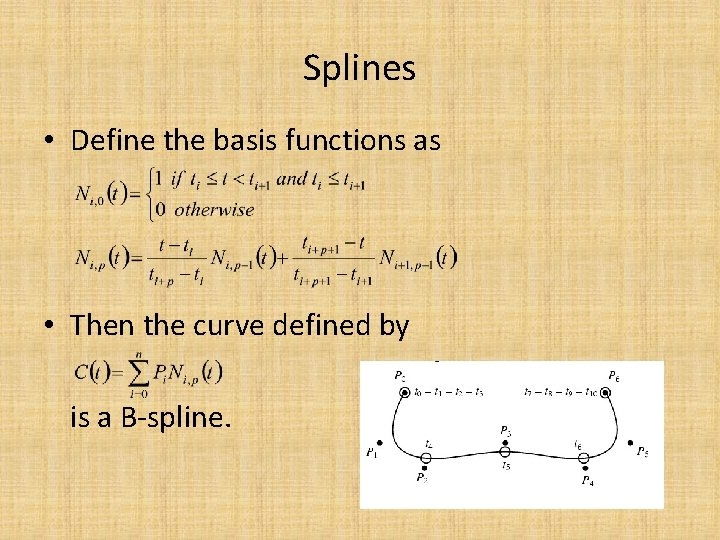Splines • Define the basis functions as • Then the curve defined by is a B-spline.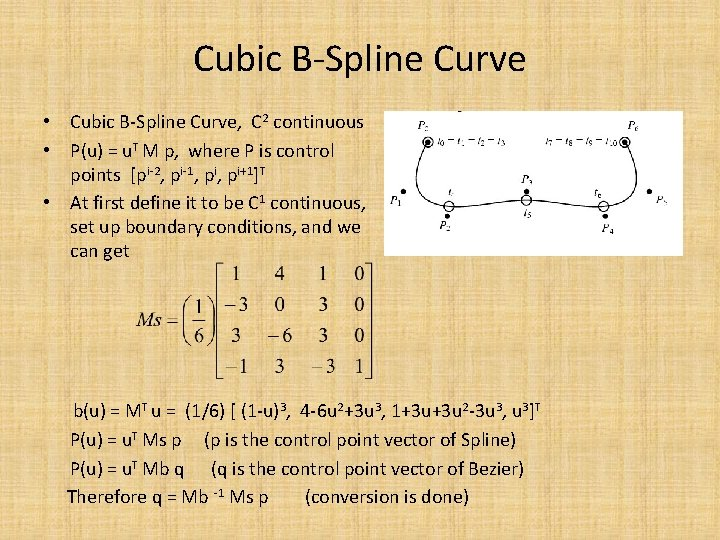Cubic B-Spline Curve • Cubic B-Spline Curve, C 2 continuous • P(u) = u. T M p, where P is control points [pi-2, pi-1, pi+1]T • At first define it to be C 1 continuous, set up boundary conditions, and we can get b(u) = MT u = (1/6) [ (1 -u)3, 4 -6 u 2+3 u 3, 1+3 u+3 u 2 -3 u 3, u 3]T P(u) = u. T Ms p (p is the control point vector of Spline) P(u) = u. T Mb q (q is the control point vector of Bezier) Therefore q = Mb -1 Ms p (conversion is done)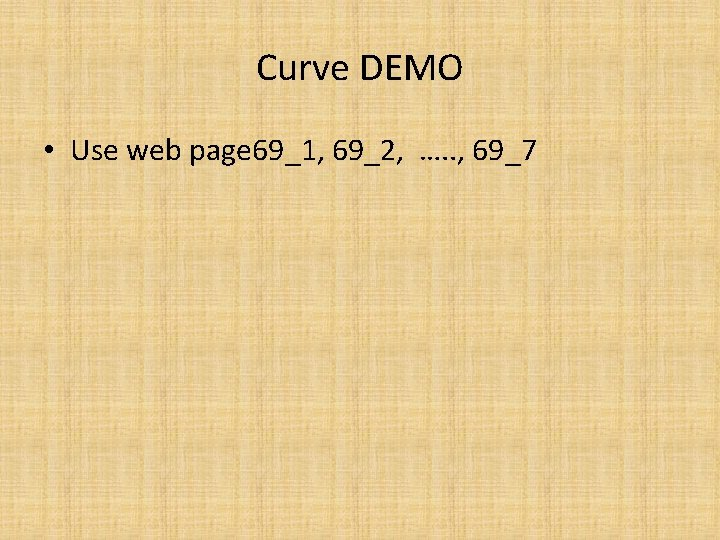Curve DEMO • Use web page 69_1, 69_2, …. . , 69_7Ray tracing: Turner Whitted • Key to success, from light to eye or from eye to screen?A simple recursive ray tracing Li: shadow ray Ri: reflected ray Ni: normal Ti: transmitted ray whether 1. L 1=R 1+T 1? or 2. f 1(L 1)=f(R 1)+f(T 1)? or 3. Color=f(L 1, R 1, T 1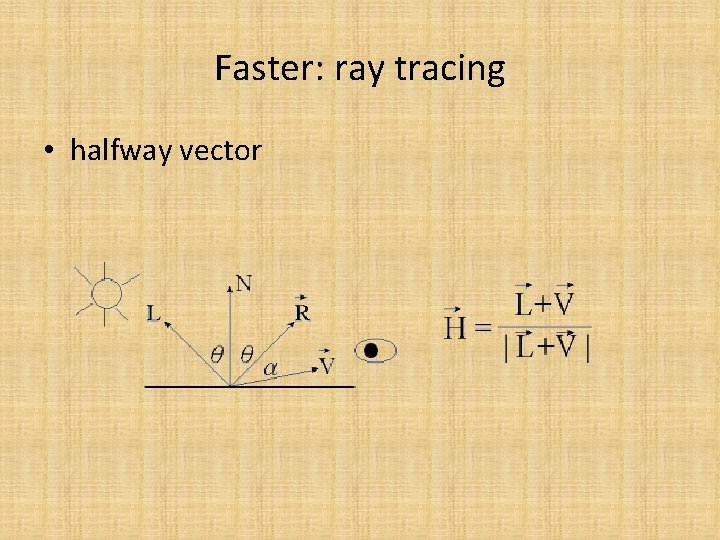Faster: ray tracing • halfway vectorFrom known data to unknown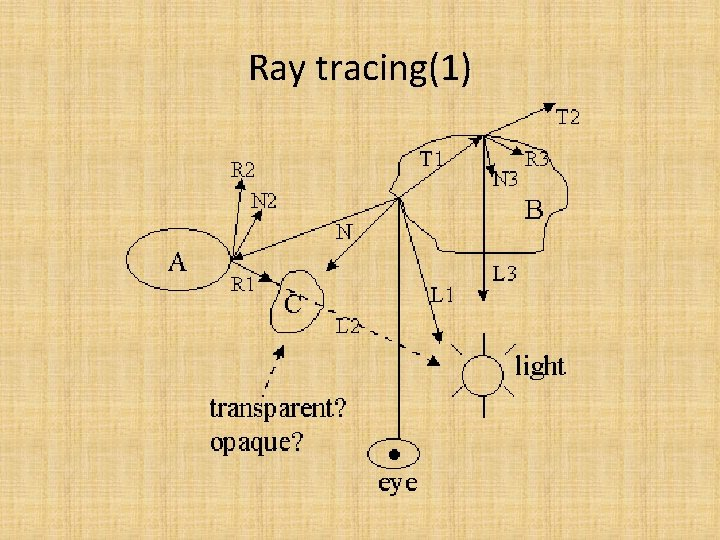Ray tracing(1)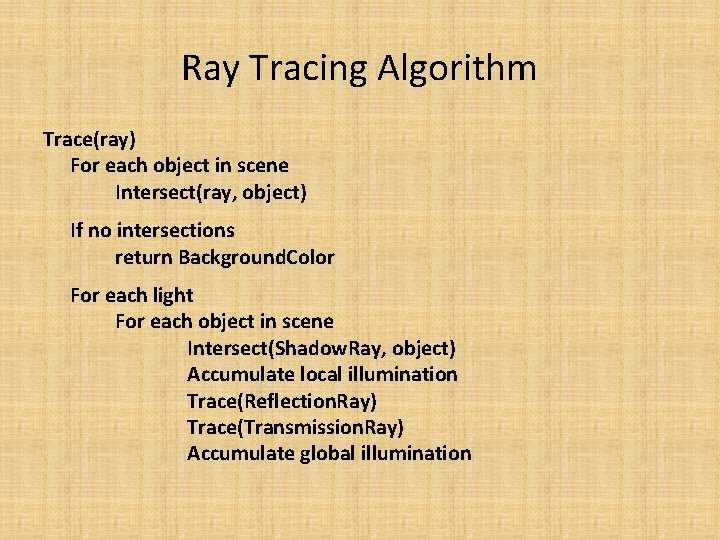Ray Tracing Algorithm Trace(ray) For each object in scene Intersect(ray, object) If no intersections return Background. Color For each light For each object in scene Intersect(Shadow. Ray, object) Accumulate local illumination Trace(Reflection. Ray) Trace(Transmission. Ray) Accumulate global illuminationRay Tracing Algorithm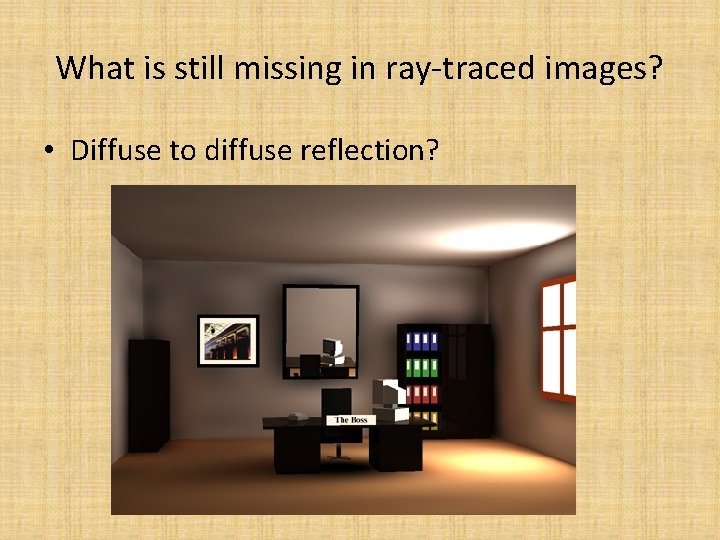What is still missing in ray-traced images? • Diffuse to diffuse reflection?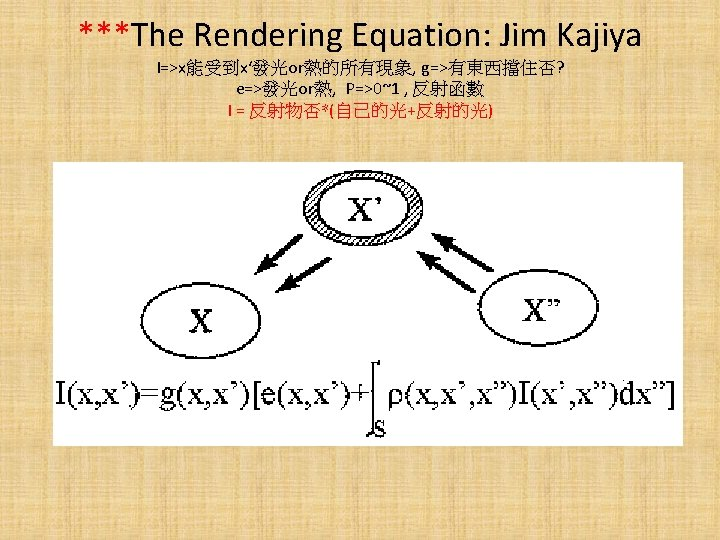Shadow in ray tracing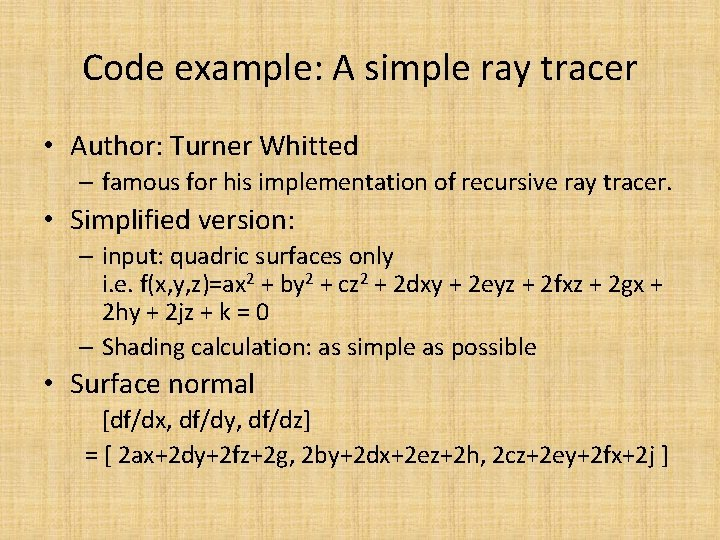Code example: A simple ray tracer • Author: Turner Whitted – famous for his implementation of recursive ray tracer. • Simplified version: – input: quadric surfaces only i. e. f(x, y, z)=ax 2 + by 2 + cz 2 + 2 dxy + 2 eyz + 2 fxz + 2 gx + 2 hy + 2 jz + k = 0 – Shading calculation: as simple as possible • Surface normal [df/dx, df/dy, df/dz] = [ 2 ax+2 dy+2 fz+2 g, 2 by+2 dx+2 ez+2 h, 2 cz+2 ey+2 fx+2 j ]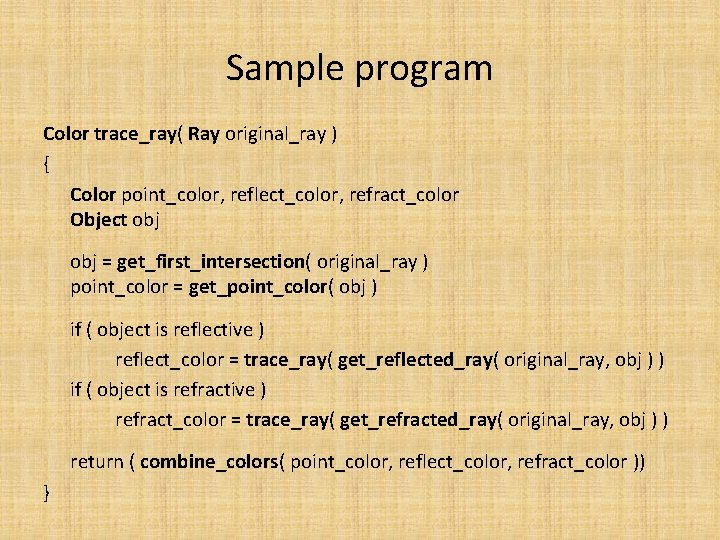Sample program Color trace_ray( Ray original_ray ) { Color point_color, reflect_color, refract_color Object obj = get_first_intersection( original_ray ) point_color = get_point_color( obj ) if ( object is reflective ) reflect_color = trace_ray( get_reflected_ray( original_ray, obj ) ) if ( object is refractive ) refract_color = trace_ray( get_refracted_ray( original_ray, obj ) ) return ( combine_colors( point_color, reflect_color, refract_color )) }Code example: A simple ray tracer • The simple ray tracer is complete and free to copy [need modification to be term project] • Input surface properties – r, g, b, relative_index_of_refraction, reflection_coef, transmission_coef, object_type – number_of_objects, number_of_surfaces, number_of_properties • How to calculate the intersection of a ray and a quadric surface?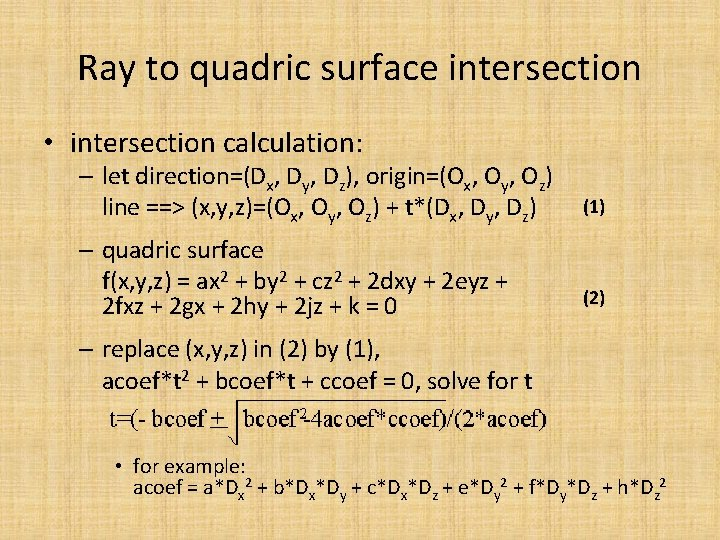Ray to quadric surface intersection • intersection calculation: – let direction=(Dx, Dy, Dz), origin=(Ox, Oy, Oz) line ==> (x, y, z)=(Ox, Oy, Oz) + t*(Dx, Dy, Dz) – quadric surface f(x, y, z) = ax 2 + by 2 + cz 2 + 2 dxy + 2 eyz + 2 fxz + 2 gx + 2 hy + 2 jz + k = 0 (1) (2) – replace (x, y, z) in (2) by (1), acoef*t 2 + bcoef*t + ccoef = 0, solve for t • for example: acoef = a*Dx 2 + b*Dx*Dy + c*Dx*Dz + e*Dy 2 + f*Dy*Dz + h*Dz 2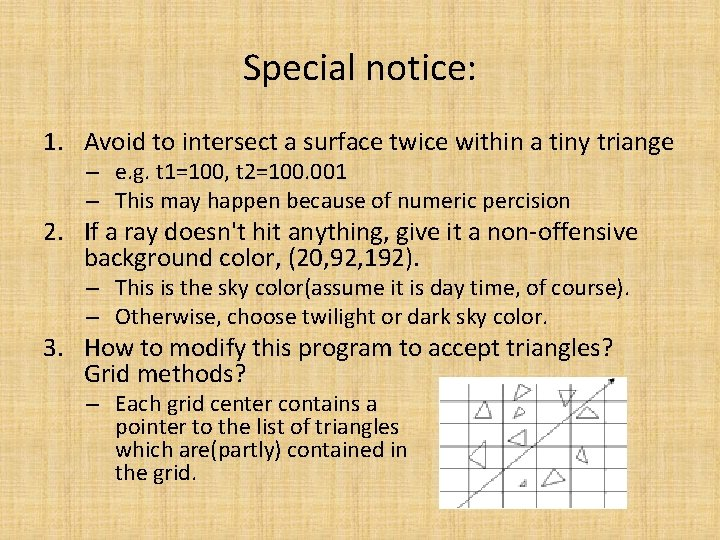Special notice: 1. Avoid to intersect a surface twice within a tiny triange – e. g. t 1=100, t 2=100. 001 – This may happen because of numeric percision 2. If a ray doesn't hit anything, give it a non-offensive background color, (20, 92, 192). – This is the sky color(assume it is day time, of course). – Otherwise, choose twilight or dark sky color. 3. How to modify this program to accept triangles? Grid methods? – Each grid center contains a pointer to the list of triangles which are(partly) contained in the grid.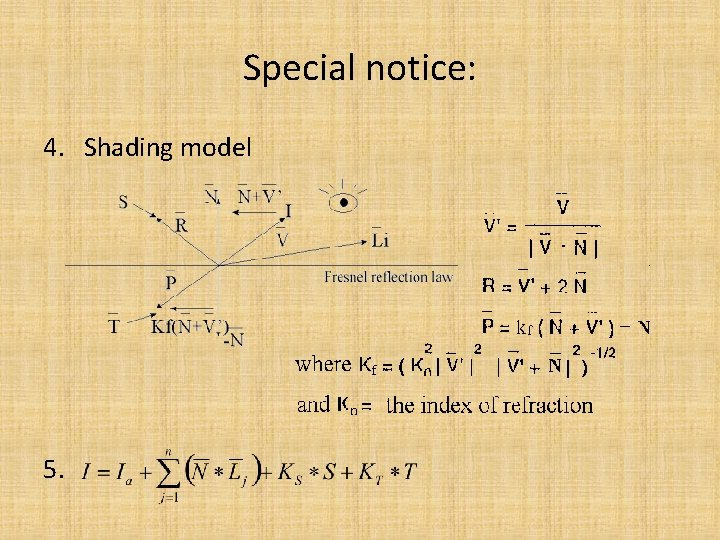Special notice: 4. Shading model 5.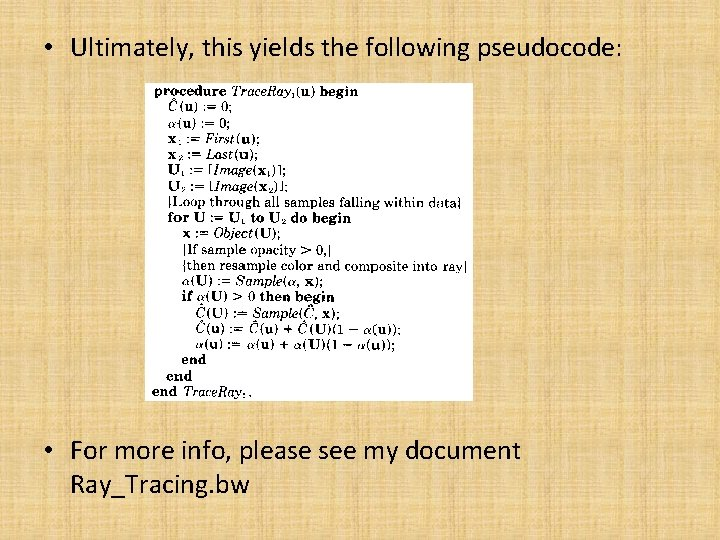• Ultimately, this yields the following pseudocode: • For more info, please see my document Ray_Tracing. bw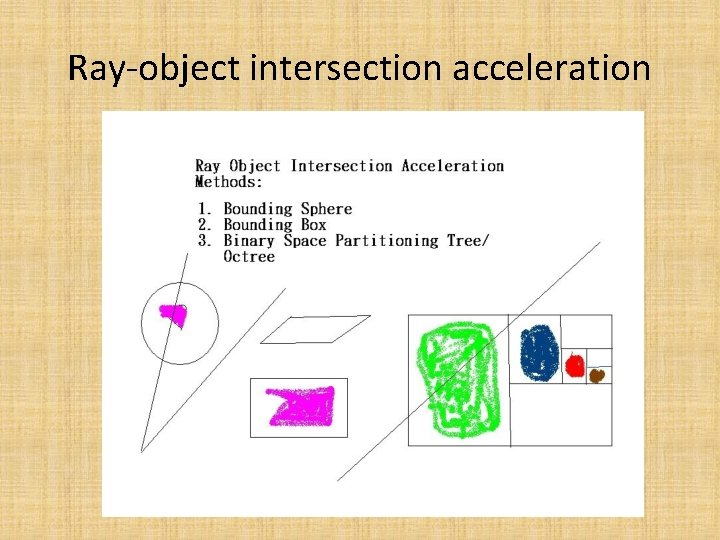Ray-object intersection acceleration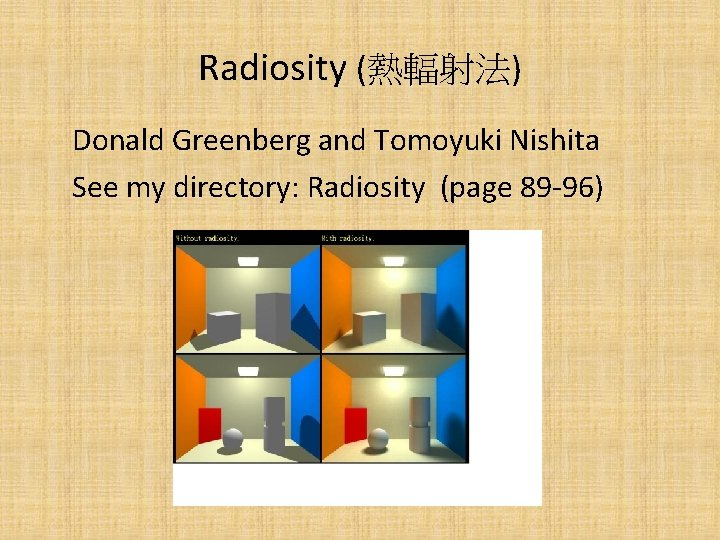Radiosity (熱輻射法) Donald Greenberg and Tomoyuki Nishita See my directory: Radiosity (page 89 -96)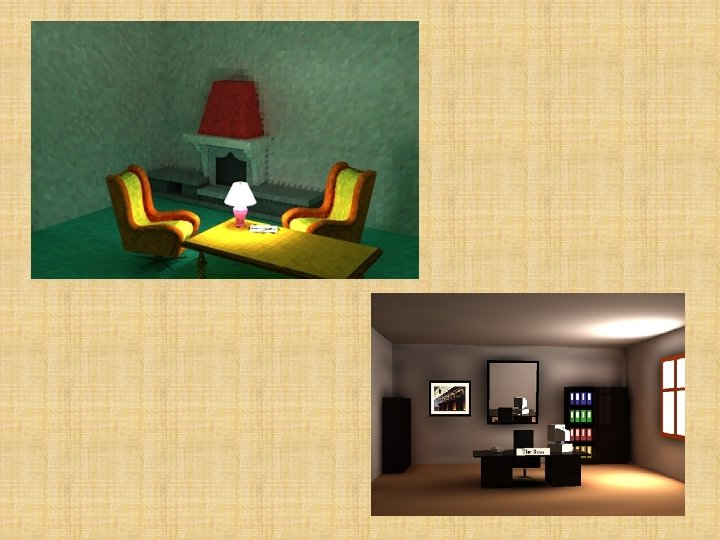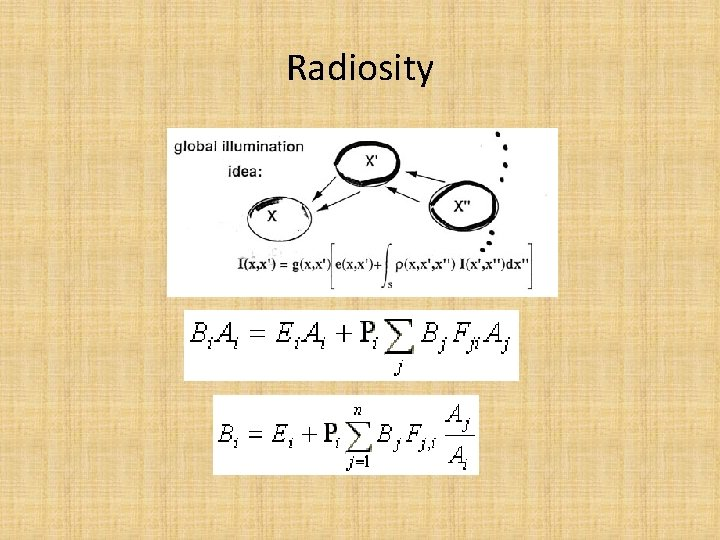Radiosity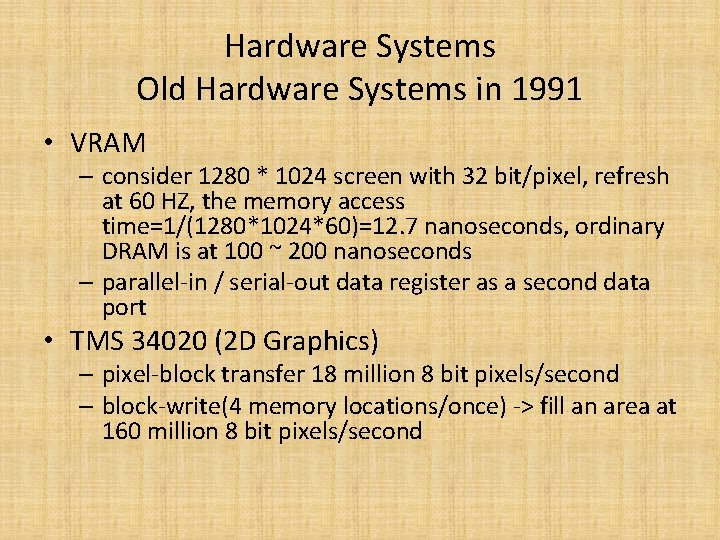Hardware Systems Old Hardware Systems in 1991 • VRAM – consider 1280 * 1024 screen with 32 bit/pixel, refresh at 60 HZ, the memory access time=1/(1280*1024*60)=12. 7 nanoseconds, ordinary DRAM is at 100 ~ 200 nanoseconds – parallel-in / serial-out data register as a second data port • TMS 34020 (2 D Graphics) – pixel-block transfer 18 million 8 bit pixels/second – block-write(4 memory locations/once) -> fill an area at 160 million 8 bit pixels/second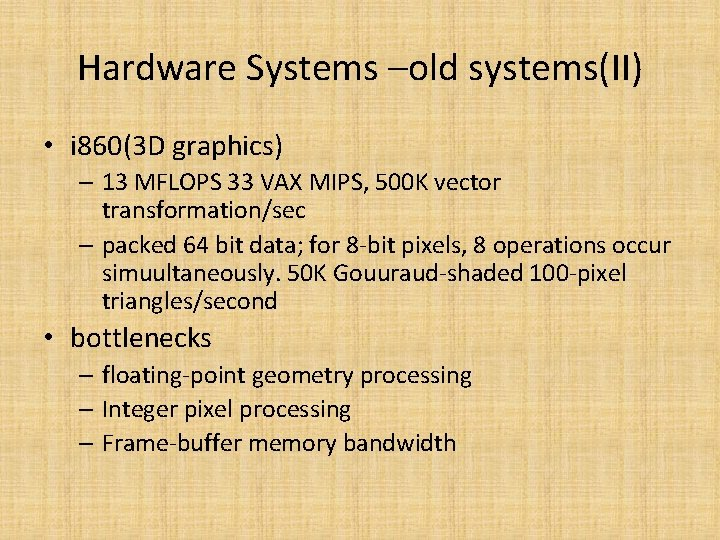Hardware Systems –old systems(II) • i 860(3 D graphics) – 13 MFLOPS 33 VAX MIPS, 500 K vector transformation/sec – packed 64 bit data; for 8 -bit pixels, 8 operations occur simuultaneously. 50 K Gouuraud-shaded 100 -pixel triangles/second • bottlenecks – floating-point geometry processing – Integer pixel processing – Frame-buffer memory bandwidth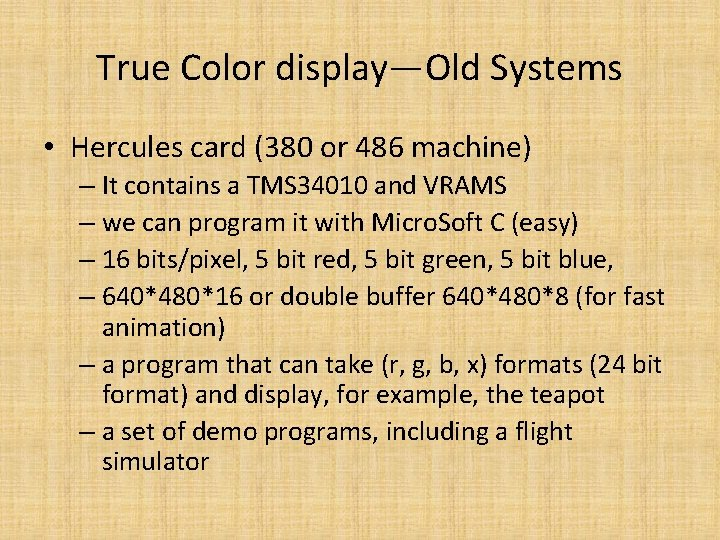True Color display—Old Systems • Hercules card (380 or 486 machine) – It contains a TMS 34010 and VRAMS – we can program it with Micro. Soft C (easy) – 16 bits/pixel, 5 bit red, 5 bit green, 5 bit blue, – 640*480*16 or double buffer 640*480*8 (for fast animation) – a program that can take (r, g, b, x) formats (24 bit format) and display, for example, the teapot – a set of demo programs, including a flight simulatorHardware system for graphics • General purpose system (MIMD: i. Warp etc) H. T. Kung • Specific system, eg: Silicon Graphics' IRIS, 4 D/240 GTX (MIMD) – 100, 000 Gouraud-shaded, Z-buffered quadrilaterals – CPU subsystem: 4 shaded-memory multiprocessors – Geometry subsystem: 5 floating-point processors, each 20 MFLOPS (Weifek 3332) – Scan-conversion subsystem: a long pipeline – Raster subsystem: 20 image engines, each for 1/20 screen, (4*5 pixel interleaved) – Display subsystem: fine graphics processor, each assigned 1/5 columns in the displayGraphics Game Machine Hardware Play. Station 2 architecture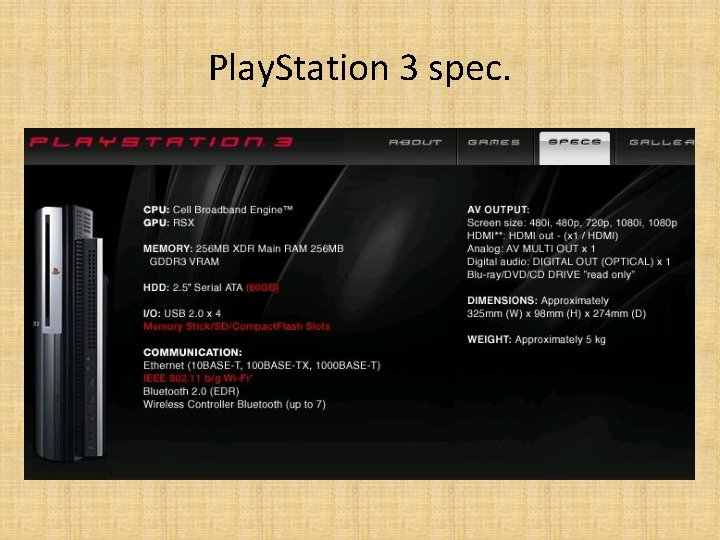Play. Station 3 spec.Play. Station 3 architecture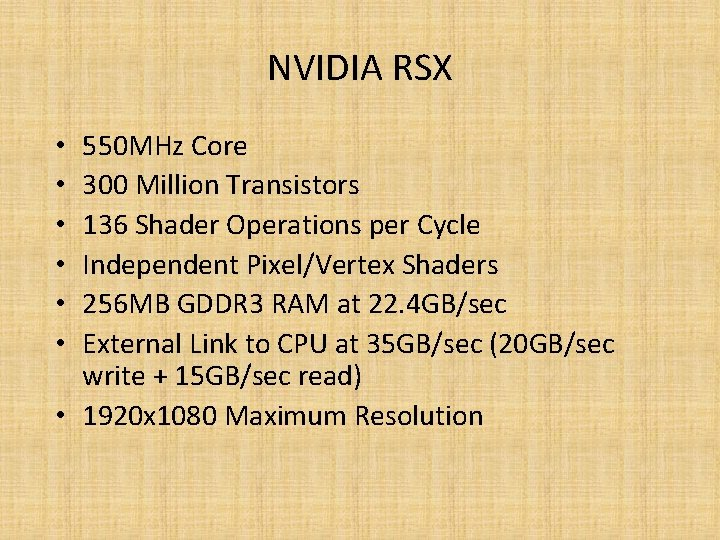NVIDIA RSX 550 MHz Core 300 Million Transistors 136 Shader Operations per Cycle Independent Pixel/Vertex Shaders 256 MB GDDR 3 RAM at 22. 4 GB/sec External Link to CPU at 35 GB/sec (20 GB/sec write + 15 GB/sec read) • 1920 x 1080 Maximum Resolution • • •ATI Radeon X 800/X 850 • (540 MHz / 1180 MHz) • 16 Pixel Pipelines (2 Vector + 2 Scalar + 1 Texture ALUs) • 6 Vertex Pipelines (1 Vector + 1 Scalar ALUs) • 92 Shader Operations per Cycle • 256 MB GDDR 3 RAM at 37. 76 GB/sec • External Link to CPU at 8 GB/secGPGPU: general purpose GPU • CUDA programming • Course by Professor Wei-Chao Chen (陳維超)